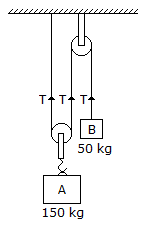# Mechanical Engineering - Engineering Mechanics

### Exercise :: Engineering Mechanics - Section 6

16.

A pendulum which executes one beat per second is known as

 A. simple pendulum B. compound pendulum C. torsional pendulum D. second's pendulum

Explanation:

No answer description available for this question. Let us discuss.

17.

Two blocks A and B of masses 150 kg and 50 kg respectively are connected by means of a string as shown in the below figure. The tension in all the three strings __________ be same.A. will B. will not

Explanation:

No answer description available for this question. Let us discuss.

18.

D' Alembert's principle basically depends upon Newton's second law of motion.

 A. Correct B. Incorrect

Explanation:

No answer description available for this question. Let us discuss.

19.

The forces, whose lines of action are parallel to each other and act in the same directions, are known as

 A. coplaner concurrent forces B. coplaner non-concurrent forces C. like parallel forces D. unlike parallel forces

Explanation:

No answer description available for this question. Let us discuss.

20.

A number of forces acting at a point will be in equilibrium, if

 A. all the forces are equally inclined B. sum of all the forces is zero C. sumof resolved parts in the vertical direction is zero (i.e. ∑V = 0) D. none of these